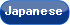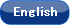Please use this identifier to cite or link to this item: `http://hdl.handle.net/10119/12301`

 Title: Sufficient completeness of parameterized specifications in CafeOBJ Authors: Nakamura, MasakiGaina, DanielOgata, KazuhiroFutatsugi, Kokichi Issue Date: 2014-12-15 Publisher: 北陸先端科学技術大学院大学情報科学研究科 Magazine name: Research report (School of Information Science, Japan Advanced Institute of Science and Technology) Volume: IS-RR-2014-005 Start page: 1 End page: 4 Abstract: CafeOBJ is a specification language which supports several kinds of specifications  . In this study, we focus on constructor-based order-sorted (CBOS) equational specifications. A signature (S,≤, Σ, Σ^C) (abbr. Σ) consists of a set S of sorts, a poset ≤ on S, a S^+ -sorted set Σ of operators, and a set Σ^C ⊆ Σ of constructors. We use the notation for the complement set Σ' = E ＼ E', constrained sorts S^ = {s ∈ S | f ∈ Σ^C_<ωs>) ∨ (f ∈Σ^C_<ωs'> ∧ s' ≤ s)}, loose sorts S^ = S ＼ S^, and constrained operators Σ^> = {f ∈ Σ_<ωs > | ω ∈ S^* ,s ∈ S^}, A specification SP is a pair of a signature Σ and a set of equations on Σ. We use the subscript A_ to refer the element of SP, e.g. S_, Σ_, E_, etc. Sufficient completeness is an important property which guarantees the existence of the initial model . A sufficient condition of sufficient completeness is given in  as follows: SP = ((S,≤, Σ, Σ^C), E) is sufficiently complete if for each S^-sorted set Y of variables of loose sorts and each term t ∈ T_<Σ ^> (Y), there exists a term u ∈ T_<Σ^C>(Y) such that t = _Eu. We call the above condition SCE. The theory of term rewriting systems (TRS) is useful to prove sufficient completeness, where equations are regarded as left-to-right rewrite rules. A term is E-reducible if it has a subterm which can be rewritten by some rewrite rule in E. It is known that the notion of basic terms is useful to show ground reducibility. We give a variant of basic terms for CBOS specifications. URI: http://hdl.handle.net/10119/12301 Material Type: publisher Appears in Collections: IS-RR-2014

Files in This Item:

File Description SizeFormat